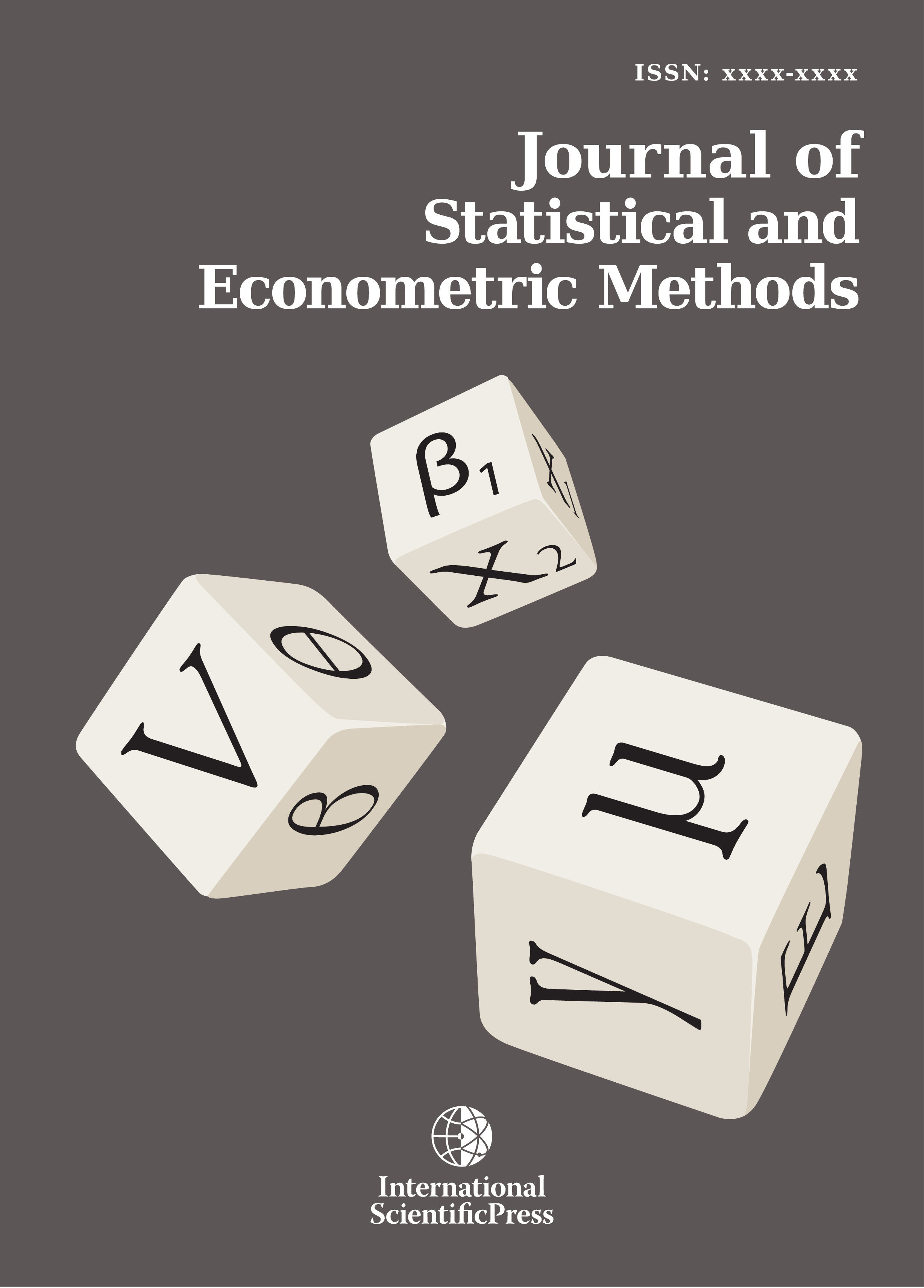# Journal of Statistical and Econometric Methods

#### The economic cost of procrastination A statistical model

•[ Download ]
• Times downloaded: 1405
• Abstract

This paper proposes a statistical model for estimating the economic cost of procrastination. The proposed model made used of given random samples on length of time of delay or procrastination by a random sample of individuals with respect to a given enterprise to calculate sample mean which was used to estimate μ , which in turn was used to estimate the parameter of any hypothesized distribution of unknown. The estimated parameters were therefore used to calculate the require probabilities, expected numbers and costs of procrastination. The model was illustrated with an example. The results showed that a salary earner of eighteen thousand naira (N18, 000.00) given the various delay time periods has an average cost of procrastination of three thousand two hundred and seventy naira twenty eight kobo (N3, 270.28) per day.ISSN: 2241-0376 (Online)
2241-0384 (Print)